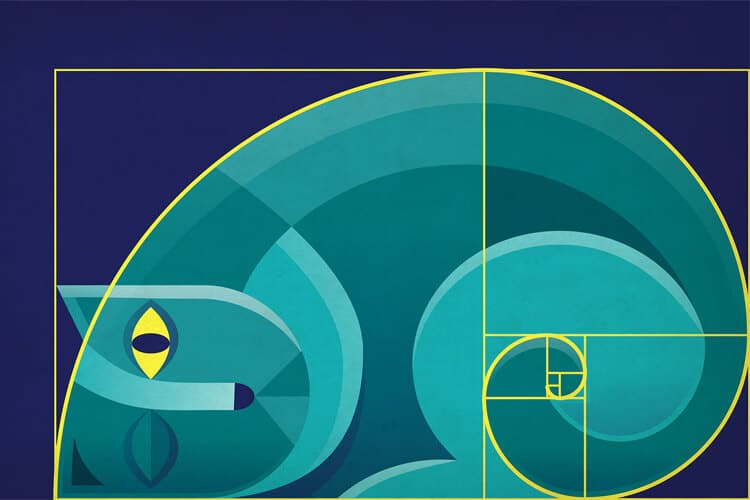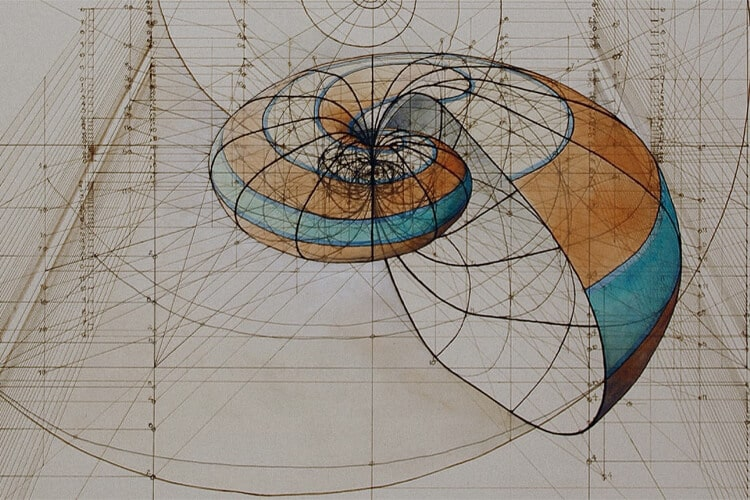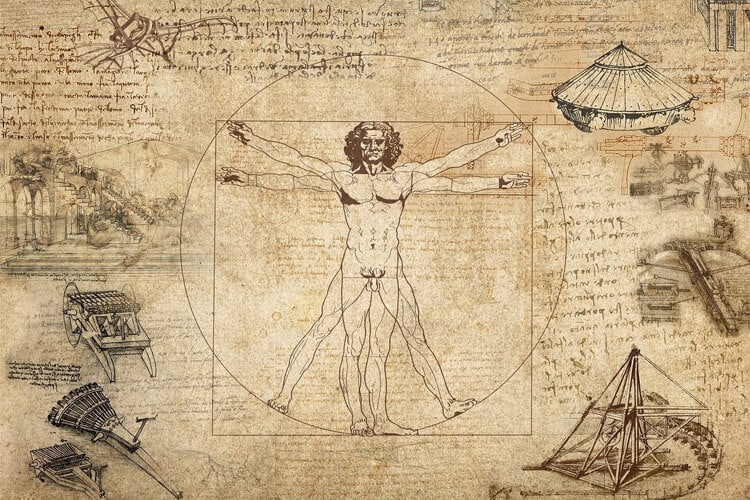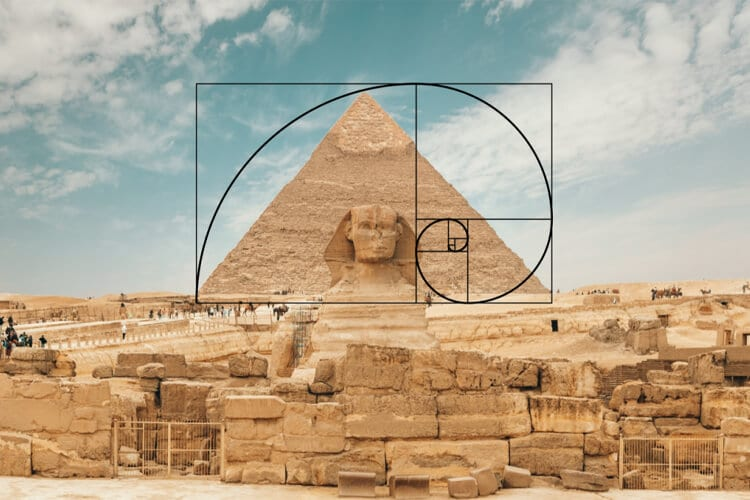# What is the Golden Ratio, the Measure of Excellence?The Golden Ratio is a term we often hear in our daily lives. We can say that this mathematical sequence, which is used in many fields from photography to painting and architecture, owes its origins to nature. In this content, we take a closer look at the Golden Ratio, which is indispensable for artists obsessed with perfection.

## What is the Golden Ratio, the Measure of Excellence? Who Found it?The Golden Ratio takes its source from the Fibonacci sequence. It is a numerical ratio relation seen in the parts that make up any object, which is thought to have the most accurate and harmonious dimensions. The sequence starts with the number 1 and proceeds after the second number, taking the sum of the two previous digits with it.

That is, the ordered sequence continues as 1, 1, 2, 3, 5, 8, 13, 21, …. At the same time, the division of each number by the previous one in this series gives a value close to 1.618 033…. As the series progresses, the ratio between the two numbers approaches the Golden Ratio. For example, the ratio of 5 to 3 is 1.666, while the ratio of 233 to 144 is 1.618.

This number, which is the symbol of the golden ratio, is referred to with the first two letters of Fibonacci’s name “fi” and denoted by the symbol “Φ”. The fact that a ratio corresponding to 1.618 has been consistently found in almost all beings since the existence of the universe has been a subject that world-renowned mathematicians have studied with admiration and used in their studies.

It may seem like an assertive statement to say that it is found in all beings. But it is possible to see this ratio in many objects around us, from the Pyramid of Cheops to the human body. Let’s take a look at the places where the golden ratio is located.

## Where is the Golden Ratio Seen?• Human Body: We can see the golden ratio in many places in our body:
• Human Head: The hair that comes out of the nodal point on our head is not linear, it comes out in a spiral. The angle of curvature of this geometric shape is the golden ratio.
• Human Face: There are many places where we can see the golden ratio on our face. For example, the ratio of the space between the eyebrows and the space between the pupils is the golden ratio. The ratio of the widths to the lengths of the front two teeth in our mouth gives 1.618.
• DNA: The DNA molecule, which carries the formula of all life, consists of two separate helixes unfolded in the vertical direction, and these helixes are 34 angstroms in length and 21 angstroms in width. The ratio of the numbers 21 and 34 to each other is the golden ratio.
• Arms: The arm, which is a part of the human body, divides the elbow into two parts, the upper and lower parts. The ratio of the area above our arms to the lower section and the ratio of the entire arms to the upper section is the golden ratio.
• Fingers: The ratio of the knuckles below the upper knuckle of your fingers and the ratio of the whole to the upper knuckle gives the golden ratio.
• The faces of famous names such as Marilyn Monroe , Alessandra Ambrosio, Katy Perry, Kelly Brook, Jessica Alba, Rita Ora, Kate Moss, Helen Mirre, Scarlett Johansson, Salma Hayek, Amber Heard (the face closest to the golden ratio) are very close to the golden ratio.

### The Golden Ratio is also frequently seen in famous structures and artifacts touched by human hands:• Egyptian Pyramids: One of the first examples that come to mind when the Golden Ratio is mentioned… The ratio of the base of each pyramid to its height is of course the golden ratio.
• Works of Leonardo da Vinci: It is impossible not to be fascinated by the golden ratio of this genius, who reflects his inclination to many sciences such as mathematics, geometry and medicine. Could it be that the ratio of the angles of all its limbs is golden, another reason why the drawing, called the Vitruvian Man, is described as the “ideal man”? His works such as “Mona Lisa”, “The Last Supper”, “Saint Jerome” were drawn in accordance with the golden ratio scheme. The ratio of the length to width of these world-famous paintings is indisputably the golden ratio. Famous names such as Botticelli, Michelangelo, Picasso and Salvador Dali also used the golden ratio abundantly in their paintings.
• Mecca and the Kaaba: A new connection is added every day between the connections between the city of Mecca, the Golden Ratio, the holy Kaaba and the Qur’an. In the measurements made with the golden ratio (Leonardo) compass, it is seen that the city of Mecca is located in the golden ratio region of Arabia, and the Kaaba is located in the golden ratio region of the city of Mecca. According to probability calculations, it is impossible for all these ratios to be random.
• Mozart Works: Studies have revealed that 6 of the 18 piano works written by Mozart fully comply with the golden ratio, and eight of them are very close to this ratio.
• Works of Mimar Sinan: The golden ratio appears in many works of the famous architect. What do you think, maybe we should attribute the splendor of the Süleymaniye and Selimiye Mosques to the golden ratio?

### Basic examples of the Golden Ratio in nature:• Pine Cone: The grains that make up the cone emerge in a spiral form from a fixed point at the bottom to the point at the top of the cone. The ratio of the curvature of these spirals is the golden ratio.
• Sea Shell: There is a curvature in the structures of these shells, and the tangent of this curvature has turned out to be the golden ratio.
• Sunflower: The ratio of the number of sunflower seeds from right to left and from left to right reveals the golden ratio.
• Daisy: The ratio of the particles moving to the right from the center of the flower and the particles going to the left is equal to the golden ratio. This is how the golden ratio is seen in almost all daisy flowers. Scientists say that this ratio ensures that the flowers get the best sunlight.
• Snow Crystals: The ratio between the short extensions and the long extensions of the crystals also gives the golden ratio.
• Tobacco Plant: The ratio of the curvature of the leaves of the Tobacco Plant also gives us the golden ratio. The same ratio applies to the fern.
• Deer Antlers: There is a golden ratio of 1.618 in the protrusions of the horns of the deer.
• Rabbit: It is possible to see the same golden ratio in the head of rabbits as in ours.
• Snail: If the Snail’s Shell is transferred to a plane, this plane creates a rectangle (golden rectangle). The ratio of the length to width of this geometric shape is the golden ratio.

Indeed, although we do not know whether there is a golden ratio on the basis of the universe we live in, or whether it is a forced way of thinking since ancient times, the only thing we can be sure of in the light of scientific data is that the universe operates according to a serious mathematical rule.

What is the Wabi-Sabi Philosophy and How Is It Applied?

What is Gothic? Origin of the Term Gothic

This post is also available in: Türkçe

Kategoriler: Art